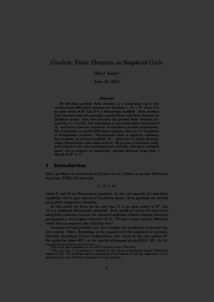Repository: Freie Universität Berlin, Math Department

# Geodesic finite elements on simplicial grids

Sander, O. (2012) Geodesic finite elements on simplicial grids. International Journal for Numerical Methods in Engeneering, 92 (12). pp. 999-1025. ISSN Online: 1097-0207Preview

407kB

Official URL: https://dx.doi.org/10.1002/nme.4366

## Abstract

We introduce geodesic finite elements as a conforming way to discretize partial differential equations for functions $v : \Omega \to M$, where $\Omega$ is an open subset of $\R^d$ and $M$ is a Riemannian manifold. These geodesic finite elements naturally generalize standard first-order finite elements for Euclidean spaces. They also generalize the geodesic finite elements proposed for $d=1$ by the author. Our formulation is equivariant under isometries of $M$, and hence preserves objectivity of continuous problem formulations. We concentrate on partial differential equations that can be formulated as minimization problems. Discretization leads to algebraic minimization problems on product manifolds $M^n$. These can be solved efficiently using a Riemannian trust-region method. We propose a monotone multigrid method to solve the constrained inner problems with linear multigrid speed. As an example we numerically compute harmonic maps from a domain in $\R^3$ to $S^2$.

Item Type: Article Mathematical and Computer Sciences > Mathematics > Numerical Analysis Department of Mathematics and Computer Science > Institute of Mathematics 1868 Ekaterina Engel 30 Mar 2016 17:49 03 Mar 2017 14:42

Repository Staff Only: item control page